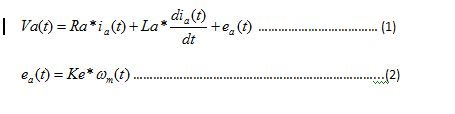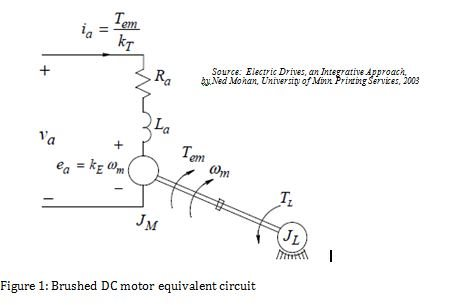# Have you considered using back-emf observers for sensorless speed estimation?

Sensorless control is gaining in popularity for motor drive applications which don’t demand precise control, below 10% rated speed. A fan or pump drives that operate within 20% to 100% speed range are the right candidates for sensorless control.

If you are internet addict like me and have ever searched “sensorless control or sensorless vector control of BLDC/PMSM motor” you would have a plethora of material. In majority of these literatures, basic methodology of sensorless motor control revolves around back-emf. From basic laws of electromagnetic theory, we know that back-emf generated inside the any electric machine’s armature winding is proportional to rotor speed.

Therefore, sensorless speed and position estimation gets divided into two basic categories:

1. Sensing or measurement of back-emf from armature terminals
2. Back-emf observer based on mathematical equations describing motor behavior

A classical example of back-emf sensing based algorithm is 120 degree commutation or trapezoidal control of brushless DC (BLDC) motor in which back-emf of non-energized phase is measured for rotor position. This helps to efficiently commutate the motor. TI's Instaspin-BLDC solution is based on this principle. A similar, yet far less common, technique can be used for DC motors also. Please refer to a helpful blog by title, “Easy cruise control for brushed motors using BEMF” based on back-emf sensing to maintain constant speed.

Closed loop back-emf observers such as sliding mode observers and/or luenberger observer rely on back-emf calculation. These techniques have their own pro’s and con’s. Frankly, it is beyond the scope of this blog to explain them in details or bring out subtle difference between them; this blog is my attempt to explain one of the basic underlying concepts which is common in all these back-emf observer based methods.

Are you thrown off by my choice of a DC motor instead of directly utilizing BLDC or PMSM motor models for explaining the underlying concept? My reasoning is pretty simple in that DC motors are the most fundamental and once you understand all its characteristics and control principles then you can apply to others. The other fact is that its armature terminal equations are simple and straight-forward which makes it much easier on me to explain the concept than dealing with complex equations of PMSM motors.

Let’s get started with DC motor equivalent circuit model as shown in figure 1, which can be represented by following two equations:Where,

Va is applied voltage at motor armature terminal, in volts

ia is armature current, in amp

Ra is armature resistance, in ohm

La is armature inductance, in henry

ea is bace-emf induced in armature, in volt

Ke is motor back-emf constant, in volt per rad per sec

ωm is motor speed in rad/secFrom equation 2, it is clear that if back-emf voltage ea is known, speed can be calculated because ke is already known parameter. To calculate back-emf voltage ea, motor equation 1 can be utilized.

Typically, DC motors are driven with pulse-width-modulated (PWM) switching power converter. There are lots of power circuit configurations which can be utilized. These range from the simple step down buck-converter consist of single MOSFET and a diode to most advanced H-bridge configuration consist of four MOSFETs and diodes.

In each case, average value of applied armature voltage Va can be very well determined based on DC link voltage and operating duty-cycle.

Now in equation 1, Ra and La is already known from the motor data sheet, Va can be determined as explained above, so if ia armature current is measured, the only unknown quantity, back-emf voltage ea can be calculated simply by subtracting the resistive and inductive drops from applied voltage Va.

Splendid, isn’t it! But, this way of calculation of back-emf voltage ea is only a mathematical possibility, it’s not a reliable solution in real world scenarios because it requires differentiation of measured current which is susceptible to measurement noise. Any induce noise in motor current measurement, such as PWM switching noise or external induced noise, will get amplified by differentiation. The result makes the back-emf calculation vulnerable to noise.

In order to avoid differentiation of measured current, closed loop observer base structure is used as shown in Figure 2 below. In the figure s is Laplace variable and 1/s represent integration.

The back-emf voltage ea, is calculated indirectly by passing the error between actual measured current and estimated current through PI controller. The idea is pretty straight forward, in order to make error between actual current and measured current to zero, the PI controller output has to become to actual back-emf ea.

In order to help you better understand this concept, I have attached simulation files based on Matlab/Simulink. The file "Parameter.m" contains motor parameter which can be used to pass user desired motor parameters to Simulink model file "Back_emf_observer.mdl".

As true with most sensorless schemes, the presented back-emf observer scheme is also sensitive to motor parameter variation especially to armature resistance. You can use these simulation files to check the estimated back-emf deviates from the actual value.

In order to reduce the sensitivity, you can try to estimate or measure the rotor temperature and do feed-forward compensation for Ra. Here it is worth to mention that with DC motors the sensitivity is worse than the BLDC/PMSM. The armature resistance of a DC motor varies much more than the stator resistance of BLDC/PMSM motors due to lake of heat-sinking capability of the rotor and obtaining right temperature from rotating object poses another challenge.

The other fact is that the advance observer schemes used with BLDC/PMSM motors are more robust and insensitive to parameter variations than mentioned scheme in which back-EMF signal reconstruction error directly contributes to the speed error.

I hope this blog provides good understanding of basic concept of sensor-less back-emf observer.

Although not so popular with DC motors, you may still consider this method with some good resistance temperature compensation schemes to minimize the speed error and meet end application requirements. For new projects on BLDC/PMSM motors using sliding mode observer, this explanation will help to correlate the similarity and give better insight on actual implementation.

TI supplies various motor control kits, which supports sliding mode observe based vector control project on C2000 based MCU. For more details, please visit control-suite.